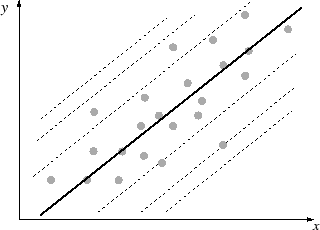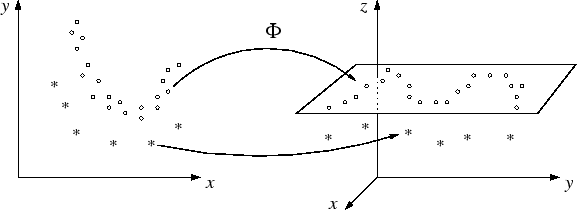Next: 5.2 Pattern association algorithm Up: 5. Kernel PCA for Previous: 5. Kernel PCA for

# 5.1 Motivation

Potential fields are used for pattern association. As for chapter 4, this was motivated by the work of Bachmann et al. (1987) and Dembo and Zeitouni (1988); however, the region of attraction is neither the set of data points nor a set of code-book vectors. Instead, this region is a subspace spanned by principal components (figure 5.1). The problem is that, usually, data are not distributed linearly. A subspace in the original data space would therefore be no good approximation of the data distribution. However, if the data are transformed into a higher-dimensional feature space, the chance is higher that in this space, the image of the data can be separated linearly from the image of its complement (Cover, 1965). Figure 5.2 illustrates this mapping. Thus, we compute the principal subspace in the feature space5.1. In the original space, the potential of a point is given by the square distance of the point's image to this subspace. Images having the same distance lie on a hyper-cylinder (here, defined as the set of points having equal distance to a subspace, for example, in three dimensions and with two principal components, the hyper-cylinder degenerates to two parallel planes). The hyper-cylinder is the iso-potential boundary of a corresponding cylindrical potential field.Next: 5.2 Pattern association algorithm Up: 5. Kernel PCA for Previous: 5. Kernel PCA for
Heiko Hoffmann
2005-03-22